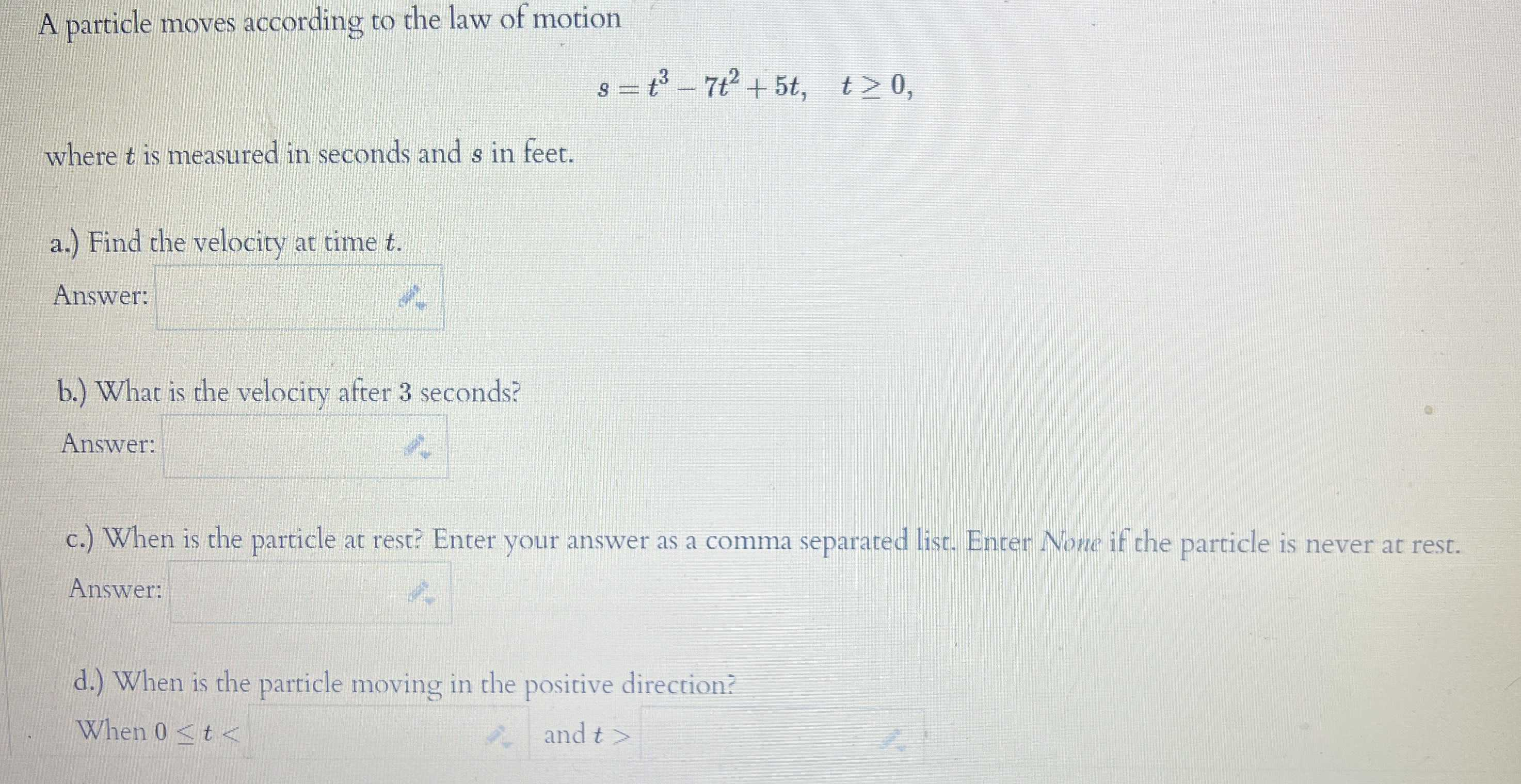### ¿Todavía tienes preguntas de matemáticas?

Pregunte a nuestros tutores expertos
Algebra
PreguntaA particle moves according to the law of motion

$$s = t ^ { 3 } - 7 t ^ { 2 } + 5 t , \quad t \geq 0$$

where $$t$$ is measured in seconds and $$s$$ in feet.

a.) Find the velocity at time $$t$$ . Answer:

b.) What is the velocity after $$3$$ seconds? Answer:

c.) When is the particle at rest? Enter your answer as a comma separated list. Enter None if the parcicle is never at rest. Answer:

d.) When is the particle moving in the positive direction?

When $$0 \leq t <$$ ___and  $$t>$$___

$$a)3t^2- 14t+ 5\\b)- 10\\c)4.27698 ,0.38968\\d)0.38968,4.27698$$